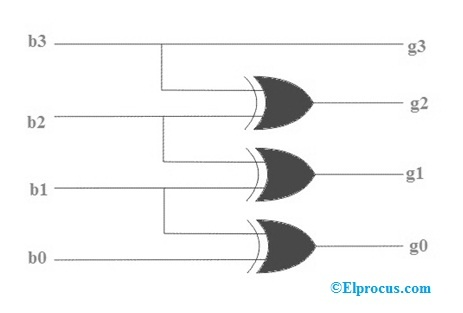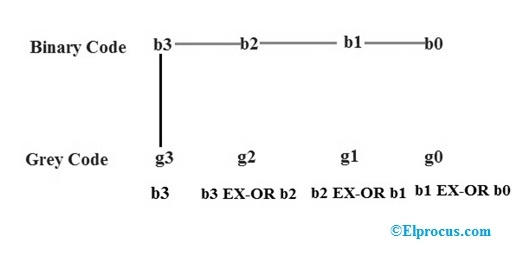# Code Converter: Binary to Gray Code and Gray Code to Binary Conversion

In computers, we need to convert binary to gray and gray to binary. The conversion of this can be done by using two rules namely binary to gray conversion and gray to binary conversion. In the first conversion, the MSB of the gray code is constantly equivalent to the MSB of the binary code. Additional bits of the gray code’s output can get using EX-OR logic gate concept to the binary codes at that present index as well as the earlier index. Here MSB is nothing but the most significant bit. In the first conversion, the MSB of the binary code is constantly equivalent to the MSB of the particular binary code. Additional bits of the binary code’s output can get using EX-OR logic gate concept by verifying gray codes at that present index. If the present gray code bit is zero then after that copy earlier binary code, as well copy reverse of earlier binary code bit. This article discusses an overview of code converters which includes binary to gray code converter as well as gray to binary code converter.

## Binary to Gray Code Converter

Binary code is a very simple representation of data using two values such as 0’s and 1’s, and it is mainly used in the world of the computer. The binary code could be a high (1) or low (0) value or else even a modify in value. Gray code or reflected binary code estimates the binary code nature that is arranged with on & off indicators, usually denoted with ones & zeros. These codes are used to look at clarity as well as error modification in binary communications.

The conversion of binary to gray code can be done by using a logic circuit. The gray code is a non-weighted code because there is no particular weight is assigned for the position of the bit. A n-bit code can be attained by reproducing a n-1 bit code on an axis subsequent to the rows of 2n-1, as well as placing the most significant bit of 0 over the axis with the most significant bit of 1 beneath the axis. The step by step gray code generation is shown below.Binary to Gray Code Conversion Logic Circuit

This method uses an Ex-OR gate to perform among the binary bits. The following best example will be very useful for knowing the conversion of binary to gray. In this conversion method, take down the MSB bit of the present binary number, as the primary bit or MSB bit of the gray code number is similar to the binary number.

To get the straight gray coded bits for generating the corresponding gray coded digit for the given binary digits, add the primary digit or the MSB digit of binary number toward the second digit & note down the product next to the primary bit of gray code, and add the next binary bit to third bit then note down the product next to the 2nd bit of gray code. Similarly, follow this procedure until the final binary bit as well as note down the outcomes depending on EX-OR logic operation to generate the corresponding gray coded binary digit.

### Example of Binary to Gray Code Converter

Let assume the Binary code digits be bo, b1, b2, b3 whereas the particular Gray Code can be attained based on the following concept.Binary to Gray Code Conversion

From the above operation, finally we can get the gray values like g3 = b3, g2 = b3 XOR b2, g1= b2 XOR b1, g0 = b1 XOR b0.Binary to Gray Code Conversion Example

For example take the binary value b3, b2, b1, b0 = 1101 and find the gray code g3, g2, g1, g0 based on the above concept

g3=b3=1

g2 = b3 XOR b2 = 1 XOR 1 =0

g1= b2 XOR b1= 1 XOR 0 = 1

g0= b1 XOR b0= 0 XOR 1 = 1

The final gray code for the value of binary 1101 is 1011

#### Binary to Gray Code Converter Table

 Decimal Number Binary Code Gray Code 0 0000 0000 1 0001 0001 2 0010 0011 3 0011 0010 4 0100 0110 5 0101 0111 6 0110 0101 7 0111 0100 8 1000 1100 9 1001 1101 10 1010 1111 11 1011 1110 12 1100 1010 13 1101 1011 14 1110 1001 15 1111 1000

## Gray to Binary Code Converter

This gray to binary conversion method also uses the working concept of EX-OR logic gate among the bits of gray as well as binary bits. The following example with step by step procedure may help to know the conversion concept of gray code to binary code.gray to Binary Code Conversion logic Circuit

To change gray to binary code, take down the MSB digit of the gray code number, as the primary digit or the MSB of the gray code is similar to the binary digit.

To get the next straight binary bit, it uses the XOR operation among the primary bit or MSB bit of binary to the next bit of the gray code.

Similarly, to get the third straight binary bit, it uses the XOR operation among the second bit or MSB bit of binary to the third MSD bit of the gray code and so on.

### Example of Gray to Binary Code Converter

Let assume the Gray Code digits g3, g2, g1, g0 whereas the particular Binary code digits are bo, b1, b2, b3 can be attained based on the following concept.Gray Code to Binary Conversion Concept

From the above operation, finally we can get the binary values like b3 = g3, b2 = b3 XOR g2, b1= b2 XOR g1, b0 = b1 XOR g0.Gray to Binary Code Conversion Example

For example take the gray value g3, g2, g1, g0 = 0011 and find the binary code b3, b2, b1, b0 based on the above concept

b3=g3=0

b2 = b3 XOR g2 = 0 XOR 0 =0

b1= b2 XOR g1= 0 XOR 1 = 1

b0= b1 XOR g0= 1 XOR 1 = 0

The final binary code for the value of gray 0011 is 0010

#### Gray to Binary Code Converter Table

 Decimal Number Gray Code Binary Code 0 0000 0000 1 0001 0001 2 0010 0011 3 0010 0011 4 0110 0100 5 0111 0101 6 0101 0110 7 0100 0111 8 1100 1000 9 1101 1001 10 1111 1010 11 1110 1011 12 1010 1100 13 1011 1101 14 1001 1110 15 1000 1111

Thus, this is all about binary to gray code converter and gray to binary code converter. From the above information finally, we can conclude that these converters play an essential role in performing different operations of digital electronics as well as communications among various number systems. The code converter examples which we have discussed in the above may helpful for understanding the concept of how to do these calculations. Here is a question for you, what are the applications of gray codes?